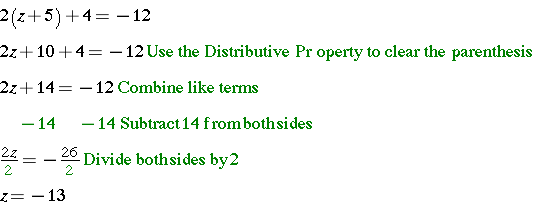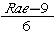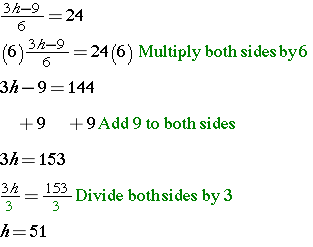# Solve Multi-Step Equations

In this lesson, you will learn to solve multi-step equations. The goal is to transform each equation into a two-step equation, which you already know how to solve. Think of solving a puzzle. The key to this puzzle is to identify how to undo all of the operations to unlock the solution!

You will use what you have learned about combining like terms and the distributive property to solve multi-step equations. Let’s look at an example of these types of equations.

### Example 1: Combining like terms to solve equationsCheck your solution: 6(6) – 1 – 3(6) = 17; 36 – 1 – 18 = 17; 35 – 18 = 17

### Example 2: Using the Distributive Property to solve equationsCheck your solution: 2 (-13 + 5) + 4 = -12; 2(-8) + 4 = -12; -16 = 4 = -12

## InvestigateRae owns three times as many baseball cards as Haley. Subtracting 9 from the number of baseball cards Rae owns and then dividing by 6 gives the number of cards Steve owns. If Steve owns 24 baseball cards, how many baseball cards does Haley own?

### Strategy

Summarize the problem, using only key words or phrases and rewrite the problem as a statement.

Find the number of baseball cards that Haley owns. (Let h represent Haley’s cards.)

Rae owns 3 times as many as Haley. (3h)

Subtracting 9 from the number of cards Rae owns and then dividing by 6 is Steve’s cards.Steve owns 24 cards.

Now, translate this information into a multi-step equation and solve.Interpret the solution: Haley owns 51 baseball cards.

(source)